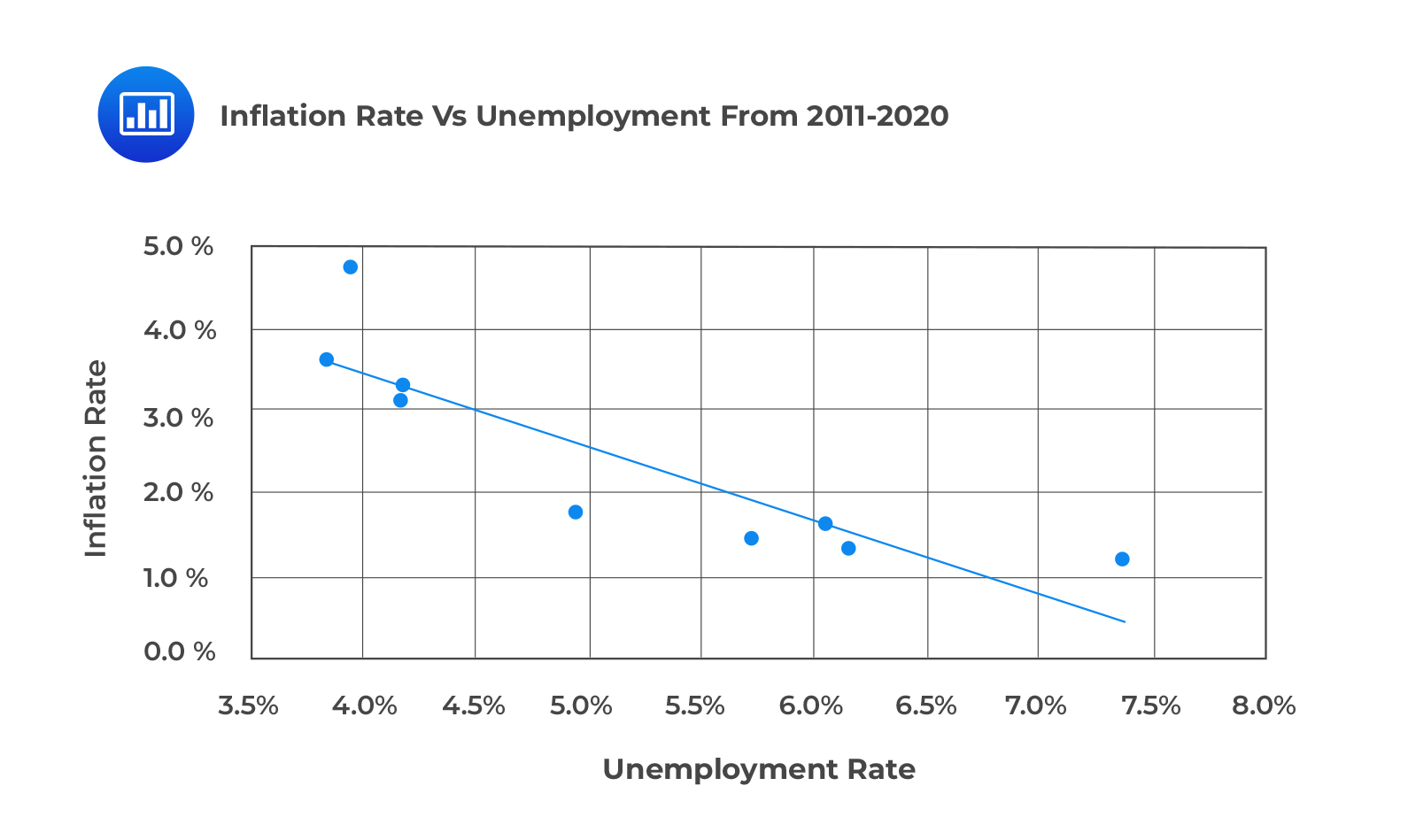# Dependent and Independent Variables

Linear regression attempts to forecast the value of a dependent variable given the value of an independent variable. It assumes that there is a linear relationship between dependent and independent variables. A Simple Linear Regression relates a dependent and one independent variable by estimating a linear relationship.

A dependent variable is a variable predicted by the independent variable. It is also known as the explained variable or endogenous variable. On the other hand, the independent variable explains the variation in the dependent variable. It is also known as the exogenous variable, explanatory variable, or predicting variable.

The following is a simple linear regression equation:

$$Y=b_0+b_1X_1+\epsilon$$

Where:

$$Y$$ = Dependent variable

$$b_0$$ = Intercept

$$b_1$$ = Slope coefficient

$$X$$ = independent variable

$$\epsilon$$ = Error term (Noise)

$$b_0$$ and $$b_1$$ are known as regression coefficients.

The error term is the part of the dependent variable that the independent variable cannot explain.

The figure below illustrates a simple linear regression model:

### Example: Dependent and Independent Variables

Artur  is using regression analysis to forecast inflation given unemployment data from 2011 to 2020.

The following table shows the relevant data from 2011 to 2020.

$$\small{\begin{array}{c|c|c}\textbf{Year} & \textbf{Unemployment Rate} & \textbf{Inflation rate}\\ \hline 2011 & 6.1\% & 1.7\%\\ \hline 2012 & 7.4\% & 1.2\%\\ \hline 2013 & 6.2\% & 1.3\%\\ \hline 2014 & 6.2\% & 1.3\%\\ \hline 2015 & 5.7\% & 1.4\%\\ \hline 2016 & 5.0\% & 1.8\%\\ \hline 2017 & 4.2\% & 3.3\%\\ \hline 2018 & 4.2\% & 3.1\%\\ \hline 2019 & 4.0\% & 4.7\%\\ \hline 2020 & 3.9\% & 3.6\%\\ \end{array}}$$

A scatter plot of the inflation rates against unemployment rates from 2011 to 2020 is shown in the following figure.What variable is the dependent variable?

#### Solution

Inflation is the dependent variable: It is the variable predicted using the unemployment rates.

## Question

The independent variable in a regression model is most likely the:

A. Predicted variable.

B. Predicting variable.

C. Endogenous variable.

#### Solution

An independent variable explains the variation of the dependent variable. It is also called the explanatory variable, exogeneous variable, predicting variable.

A and C are incorrect. A dependent variable is a variable predicted by the independent variable. It is also known as the predicted variable, explained variable, endogenous variable.

Reading 0: Introduction to Linear Regression

LOS 0 (a) Describe a simple linear regression model and the roles of the dependent and independent variables in the model

Shop CFA® Exam Prep

Offered by AnalystPrepLevel I
Level II
Level III
All Three Levels
Featured Shop FRM® Exam PrepFRM Part I
FRM Part II
FRM Part I & Part II
Learn with Us

Subscribe to our newsletter and keep up with the latest and greatest tips for success
Shop Actuarial Exams PrepExam P (Probability)
Exam FM (Financial Mathematics)
Exams P & FMGMAT® Complete CourseDaniel Glyn
2021-03-24
I have finished my FRM1 thanks to AnalystPrep. And now using AnalystPrep for my FRM2 preparation. Professor Forjan is brilliant. He gives such good explanations and analogies. And more than anything makes learning fun. A big thank you to Analystprep and Professor Forjan. 5 stars all the way!michael walshe
2021-03-18
Professor James' videos are excellent for understanding the underlying theories behind financial engineering / financial analysis. The AnalystPrep videos were better than any of the others that I searched through on YouTube for providing a clear explanation of some concepts, such as Portfolio theory, CAPM, and Arbitrage Pricing theory. Watching these cleared up many of the unclarities I had in my head. Highly recommended.Nyka Smith
2021-02-18
Every concept is very well explained by Nilay Arun. kudos to you man!2021-02-13